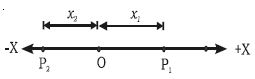# Motion in One Dimension or Rectilinear Motion

The motion along a straight line is known as rectilinear motion. The important parameters required to study the motion along a straight line are the position, displacement. velocity, and acceleration.

Position, displacement and distance travelled by the particle

The motion of a particle can be described if its position is known continuously with respect to time. The total length of the path is the distance travelled by the particle and the shortest distance between the initial and final position of the particle is the displacement. The distance travelled by a particle. however, is different from its displacement from the origin.Fig: Distance and Displacement

For example, if the particle moves from a point O to position P1 and then to position P2, its displacement at the position P2 is – x2 from the origin but, the distance travelled by the particle is x1 + x1 + x2 = (2x1 + x2) (Fig). The distance travelled is a scalar quantity and the displacement is a vector quantity.